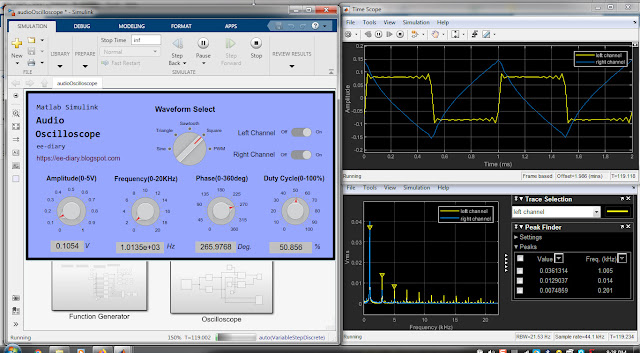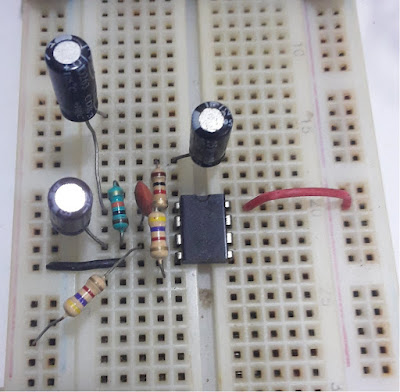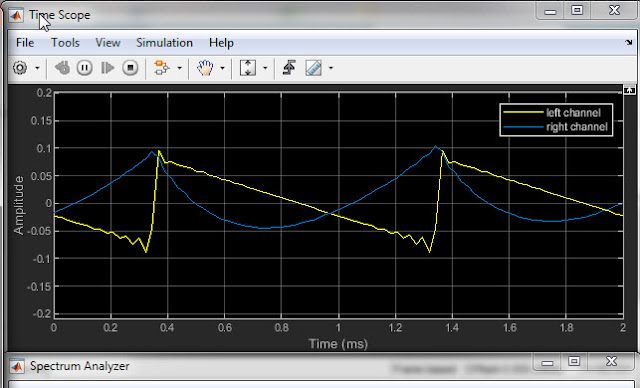# LM358 Op-Amp Integrator Test with Matlab Simulink Oscilloscope

In this test experiment, it is shown how LM358 op-amp based integrator works and how it can be tested with Matlab Simulink oscilloscope, waveform generator and spectrum analyzer. It is shown with the help of matlab and simulink, the various response from the integrator circuit to different type of inputs. The test results of the experiment are provided.

### Matlab/Simulink Oscilloscoe, Waveform Geneator and Spectrum Analyzer

The function generator which is capable to generate sine wave, sawtooth wave, triangle wave, PWM wave and square wave was build with the Matlab GUI application builder. The function generator can generate signals within audio frequencies and is sufficient to test working of electronics circuit like op-amp integrator.It also has two channels, right and left, so two independent signal can be fed into the circuit being tested. Similarly, the oscilloscope in Simulink enables us to test the designed circuit in real time, perform signal analysis in time domain. Also the Simulink spectrum analyzer allows us to perform frequency analysis of the input and output signal from the op-amp circuit and also perform frequency response analysis of the op-amp integrator.

The following shows the signal generator, oscilloscope and spectrum analyzer in Matlab and Simulink.### LM358 op-amp integrator

An op-amp integrator perform summation over a period of signals and can be used for solving differential equation. Here it build using LM358 op-amp with single power supply interface. When used in combination with differentiator, summing amplifier and gain blocks analog computer can be build. Such analog computer does not require any programs to run and they act in real time. Integrator op-amp circuit is also used for waveform shaping and is used in function generator.

A simple op-amp integrator circuit is shown below.

Since the inverting terminal input is at virtual ground, we have using KVL,

$$V_c + V_{out} = 0$$

or, $$V_{out}(t) = -V_c(t)$$  ------------>(1)

That is, the output voltage is equal to the capacitor voltage,

Also due to virtual ground, all input voltage drops across the input resistor and from Ohm's law we have,

$$i(t)=\frac{V_{in}(t)}{R_i}$$  ---------------->(2)

The capacitor current and capacitor voltage are related by,

$$i(t)=C\frac{dv(t)}{dt}$$    -------------->(3)

and so, taking integration we get,

$$V_c(t)=\frac{1}{C}\int_{0}^{t}{i(t)}\,dt$$  -------------->(4)

Ans so using equation(2) we have,

$$V_c(t)=\frac{1}{R_iC}\int_{0}^{t}{V_{in}(t)}\,dt$$  ------------->(5)

And using equation (1), the output voltage is,

$$V_{out}(t)=-\frac{1}{R_iC}\int_{0}^{t}{V_{in}(t)}\,dt$$  -------------->(6)

Equation (6) tells that the output voltage is the integration of the input signal over period t. Later we will see the response of the integrator circuit when the input signal is square wave, sine wave, triangle wave etc.

### LM358 op-amp integrator on breadboard

The LM358 op-amp supports single power supply interface so here the single power supply is used. The op-amp integrator circuit is build on a breadboard. Picture below show the LM358 op-amp integrator circuit which is build on a breadboard.Here although electrolytic capacitor was used, a better choice for integrator circuit is to use polypropylene film capacitor because they are relatively stable and have low-leakage.

### LM358 op-amp integrator circuit diagram

The circuit diagram of the LM358 op-amp integrator using +5V single supply is shown below.

### Testing & Results

The LM358 integrator above was tested with various type of signals from the function generator. Response of the integrator to various amplitude and frequencies was acquired and analyzed. The following are some of the results.

First square wave input was tested. If the input to an integrator is a square wave then the output will be triangle wave. See also the tutorial Square wave generator using LM358. The following shows the input and output waveform of the integrator displayed on the time scope

The spectrum analyzer shows the frequencies information of the input and output as shown below for 1KHz input.

If the input to an integrator is triangle wave then the output is a sine wave which is shown below.

If the input is a sine wave then we get 180 phase shifted signal which is a cosine wave. This is shown below.

The above three inputs are utilized in many analog circuits such as creating a function generator.

With the matlab/simulink function generator we can also send in sawtooth wave and PWM wave.

The following shows the output when the input is a sawtooth wave to the integrator.The following shows 80% duty cycle PWM(Pulse Width Modulation) input and output from LM358 integrator circuit.

### Video demonstration

The following is video demonstration of testing the LM358 op-amp integrator on breadboard with Matlab/Simulink oscilloscope.